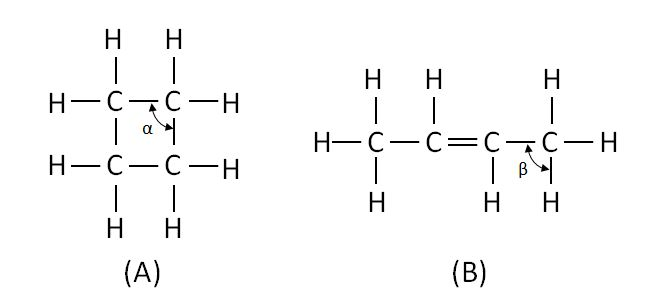# Cyclobutane and ButeneThe above are the structural formulas of two compounds which have the same molecular formula. Which of following statements is correct?

a) The bond angle $\alpha$ is the same as the bond angle $\beta$.

b) Compound (A) is a saturated hydrocarbon and compound (B) is an unsaturated hydrocarbon.

c) The bond length between the carbon atoms is identical for all bonds.

d) None of the above

×